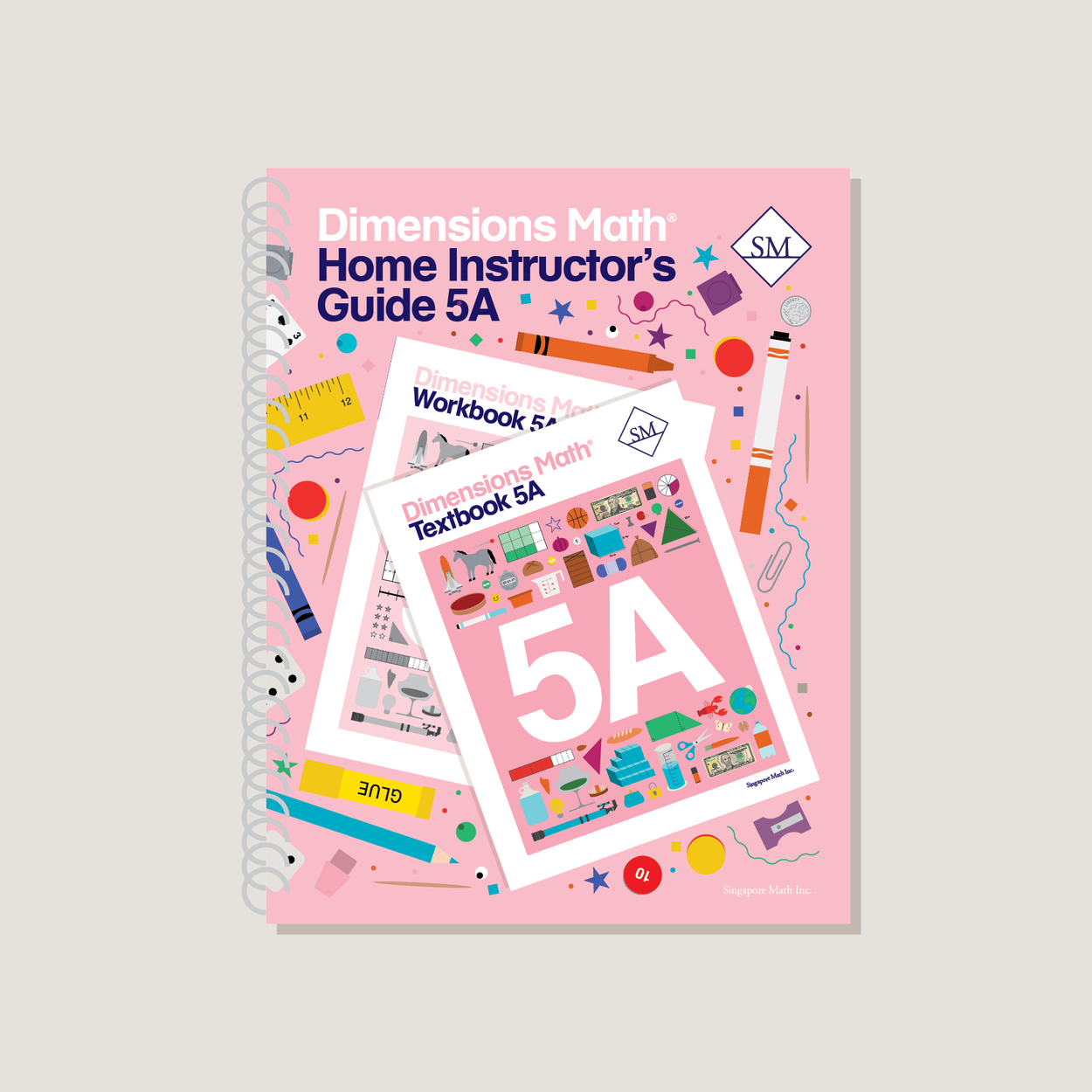# Dimensions Math Home Instructor's Guide 5A

\$21.40

Dimensions Math Home Instructor’s Guides are an essential resource for teaching Dimensions Math in a home or one-on-one setting. The purpose of the Guides is to help educators understand concepts in the Dimensions Math curriculum and teach as effectively as possible. They include extensive background notes, lesson suggestions, tips, and activities. Home Instructor’s Guides include answer keys for corresponding Dimensions Math Textbooks and Workbooks.

Note: Soft cover, spiral bound.

SKU: DMHIG5A

ISBN: 9781947226661

Pagecount: 224

Dimensions: 8.5 x 11 x 0.5 in

Chapter 1: Whole Numbers
Lesson 1: Numbers to One Billion
Lesson 2: Multiplying by 10, 100, and 1,000
Lesson 3: Dividing by 10, 100, and 1,000
Lesson 4: Multiplying by Tens, Hundreds, and Thousands
Lesson 5: Dividing by Tens, Hundreds, and Thousands
Lesson 6: Practice

Chapter 2: Writing and Evaluating Expressions
Lesson 1: Expressions with Parentheses
Lesson 2: Order of Operations — Part 1
Lesson 3: Order of Operations — Part 2
Lesson 4: Other Ways to Write and Evaluate Expressions
Lesson 5: Word Problems — Part 1
Lesson 6: Word Problems — Part 2
Lesson 7: Practice

Chapter 3: Multiplication and Division
Lesson 1: Multiplying by a 2-digit Number — Part 1
Lesson 2: Multiplying by a 2-digit Number — Part 2
Lesson 3: Practice A
Lesson 4: Dividing by a Multiple of Ten
Lesson 5: Divide a 2-digit Number by a 2-digit Number
Lesson 6: Divide a 3-digit Number by a 2-digit Number — Part 1
Lesson 7: Divide a 3-digit Number by a 2-digit Number — Part 2
Lesson 8: Divide a 4-digit Number by a 2-digit Number
Lesson 9: Practice B

Chapter 4: Addition and Subtraction of Fractions
Lesson 1: Fractions and Division
Lesson 3: Subtracting Unlike Fractions
Lesson 4: Practice A
Lesson 5: Adding Mixed Numbers— Part 1
Lesson 6: Adding Mixed Numbers— Part 2
Lesson 7: Subtracting Mixed Numbers— Part 1
Lesson 8: Subtracting Mixed Numbers— Part 2
Lesson 9: Practice B

Chapter 5: Multiplication of Fractions
Lesson 1: Multiplying a Fraction by a Whole Number
Lesson 2: Multiplying a Whole Number by a Fraction
Lesson 3: Word Problems — Part 1
Lesson 4: Practice A
Lesson 5: Multiplying a Fraction by a Unit Fraction
Lesson 6: Multiplying a Fraction by a Fraction — Part 1
Lesson 7: Multiplying a Fraction by a Fraction — Part 2
Lesson 8: Multiplying Mixed Numbers
Lesson 9: Word Problems — Part 2
Lesson 10: Fractions and Reciprocals
Lesson 11: Practice B
Review 1

Chapter 6: Division of Fractions
Lesson 1: Dividing a Unit Fraction by a Whole Number
Lesson 2: Dividing a Fraction by a Whole Number
Lesson 3: Practice A
Lesson 4: Dividing a Whole Number by a Unit Fraction
Lesson 5: Dividing a Whole Number by a Fraction
Lesson 6: Word Problems
Lesson 7: Practice B

Chapter 7: Measurement
Lesson 1: Fractions and Measurement Conversions
Lesson 2: Fractions and Area
Lesson 3: Practice A
Lesson 4: Area of a Triangle — Part 1
Lesson 5: Area of a Triangle — Part 2
Lesson 6: Area of Complex Figures
Lesson 7: Practice B

Chapter 8: Volume of Solid Figures
Lesson 1: Cubic Units
Lesson 2: Volume of Cuboids
Lesson 3: Finding the Length of an Edge
Lesson 4: Practice A
Lesson 5: Volume of Complex Shapes
Lesson 6: Volume and Capacity — Part 1
Lesson 7: Volume and Capacity — Part 2
Lesson 8: Practice B
Review 2

A & B Books: Our programs divide the school year into two semesters. “A” level books are for the first half of the school year. “B” level books are for the second half of the school year. You need both “A” and “B” material for a complete school year.

Required Components: Textbooks, Workbooks, and Guides (either Home Instructor’s Guides or Teacher’s Guides) are all necessary components. These three elements each serve a unique function and work together to build math mastery.

Printouts: Dimensions Math PK-5 Resources

Recommended Manipulatives: Dimensions Math Grades PK-5 Recommended Manipulatives